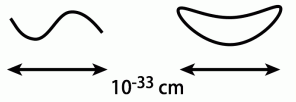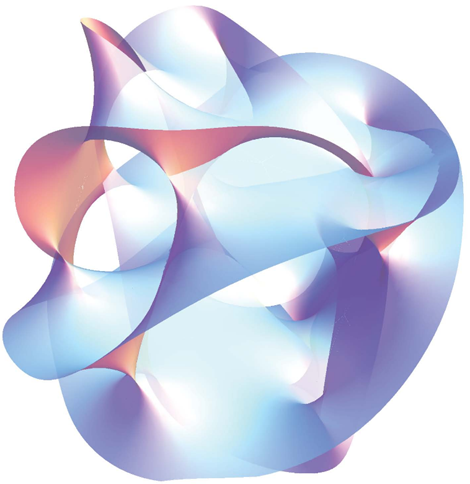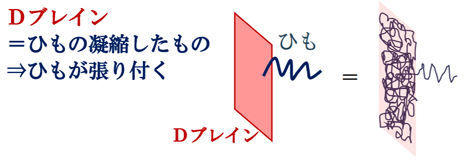# ２４　Superstring Theory：a Theory of Quantum Gravity　#StringTheory #universe

２４　Superstring Theory：a Theory of Quantum Gravity　#StringTheory #universe

[Electromagnetic force + Weak interaction＋Strong interaction ＋ Gravity]
○The phenomenon and force in the microscopic world is can be explained by the quantum theory, and macroscopic gravity phenomenon is can be explained by the general theory of relativity.
○Since 1980, scientists around the world are working on the development of the superstring theory that unifies general theory of relativity and the quantum theory, and that theory can be explained from the microscopic to the macroscopic world.
○Quantum theory has poor compatibility with gravity, would have obtained a result of the contradiction to be fitting the quantum theory to gravity. Superstring theory is expected as candidates for the theory that can be understood gravity in the framework of the quantum theory.

○For example, if the (early) size of the universe is as small as a point, the energy becomes infinite. The point of this energy infinity is the singularity.
○In the singularity the space-time disappears, and all of the physical law does not hold.
○If the particle is made of a string as shown by the string theory, the singularity does not come out.

○The superstring theory can handle gravity like the other three forces by representing the elementary particles as vibrations of the strings.
※In quantum scale, basic strings have become closed loope. Althoug the strings have various excited states, in one of such excited states, there is one with the nature of graviton(:particle that mediate gravity).※Open string and closed string 

○In addition, the theory is possible to combine fermions and bosons together by thinking of super symmetry.
○However, considering that there is super-symmetry, it is necessary to assume the partner particles obtained by inverting particles and antiparticles, as a result, the number of particles are more than 100 kinds. Moreover, the space is a 10 dimensional.
※When trying to describe various particles uniformly, it becomes a theory of high dimension and supersymmetry. And with supersymmetry, there is only 11 –dimensional theory at maximum.

○Spatial dimension of 5-10（Extra Dimension：Calabi–Yau manifold） are folded into very fine "Planck distance" (10^-35 m) of magnitude. Various properties of elementary particles come out from there.
※Since there is no dimension of time in the extra 6-dimensional part of the string theory, the Euclidean space is bent and the gauge symmetry is naturally generated.
※Extra Dimension：Calabi–Yau manifold※Gauge symmetry: If there is a common physical law between the physical law of a certain world and the physical law of another world, a conversion rule of rulers is required. The fact that the physical law is established with this measure (gauge) conversion rule is called "gauge symmetry".

○By using string theory, we can calculate the entropy of black hole, Hawking radiation and so on. 

○Black hole entropy 
・Very heavy substances are required to make black holes.
・In string theory, D-brane is a good candidate.
・D-brane = a condensed one of strings
・Strings stick to D -brane .・Black hole＝D –brane＋Assembly of strings
・The number of string states matches the expected entropy.

○However from the size of a "string" which this theory assumes being very small(10^－35m) etc., the superstring theory has not acquired the status as an established theory of physics.

＜Multiverse (Multi-Universe)＞ 
・Superstring theory suggests that the Big Bang there was many times. In other words, the universe is not one. It will be that the universe is multi and there are parallel universes.
• In the parallel universe, the laws of physics are different, but superstring theory's seems applicable.

・There is no experimental evidence to date that confirms string theory's predictions of supersymmetry and Kaluza–Klein extra dimensions. 
• Verification of superstring theory by accelerator is not realistic. Superstring theory may be useful for clarifying black holes and dark matter.

【References】
1. A Universe from Nothing. Lawrence M. Krauss -2012
2. “超弦理論とブレーン世界”at ILCの物理（Japanese）
3. String theory - Wikipedia
4.Black hole thermodynamics - Wikipedia
5. 高柳 匡” 超ひも理論の最前線： 宇宙は量子ビットから 創られているのか？“20170524
6.Michio Kaku / New York incandescent classroom "Dream of Einstein " 20150403
7. Ｎｏ．２８－１－１　Superstring theory and Brane cosmology
8. Loop Quantum Gravity - Wikipedia

【Change log】
20170401　 Addition of figure of strings
20170409 Addition of figure of Extra Dimension and Digression
20171229　Addition of Black hole entropy